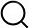# Also in the Article

Acquisition and Analysis of Line Scans.
This protocol is extracted from research article:
Visualization of β-adrenergic receptor dynamics and differential localization in cardiomyocytes
Proc Natl Acad Sci U S A, Jun 4, 2021;

Procedure

Two methods were applied and compared to extract the behavior of the diffusing species, namely FCS and STICS.

In the first approach, temporal autocorrelation in each pixel of the line scan was calculated. From the recorded fluorescent intensity time trace arising from the detection volume, a temporal autocorrelation G(τ), which reflects the timescale of the fluorescence intensity fluctuations, can be calculated according to the following formula:

The pointed brackets represent an averaging over all time values t.

By including photophysical processes, the following equations were derived to fit the calculated autocorrelation functions (58):

in which γ is a constant shape factor for the excitation volume, ω0 is the distance in the image plane where the excitation volume intensity decays to the value I(R,Z)/I0 = e−2, D is the diffusion constant, N is the number of particles, τ is the temporal time lag, τT is the lifetime of the photophysical process, T is the fraction of molecules in this process, and G is the limiting value of G(τ) for τ → ∞ (33).

The STICS analysis performs a global spatial and temporal analysis on all pixels and times of the line-scan kymograph, yielding a spatiotemporal correlation function. Such two-dimensional plots, or STICS functions, have two axes and display the process of diffusion in a shape of a “plume,” which broadens in space as a function of time. In STICS, the spatiotemporal image correlations of these line scans are calculated, circumventing the influence of photobleaching (32). The kymographs are corrected for drifts and slow fluctuations using a random number addition detrending within a moving window of ∼250$⋅103$ lines (about 20 s) similar to ref. 40. The spatiotemporal correlation curve is calculated:

where $〈〉$ indicates the average over all positions x and scans i (corresponding to time ti) and $δIx,ti=Ix,ti−⟨Ix,ti⟩$. ξ represents the spatial lag variable, ti = iT is the time, and τi = iT is the discrete time lag as an integer multiple of the scanning period. Alternatively, by calculating the fast Fourier transform and its complex conjugate, the autocorrelation or STICS function was determined by performing the inverse fast Fourier transform of the product of the two transforms (31).

The spatiotemporal correlation curve for pure diffusion in a one-dimensional line scan can then be fitted to the following fitting model (31, 32):

From horizontal cross-sections of the STICS function, the different time lags of the average MSD can be extracted. From these plots at time τ = 0, the waists of the PSF can be extracted. Further details on the data analysis strategy to extract both the STICS plots as well as the single-point autocorrelation curves can be found in SI Appendix, Supplementary Materials and Methods.

Note: The content above has been extracted from a research article, so it may not display correctly.

Q&A